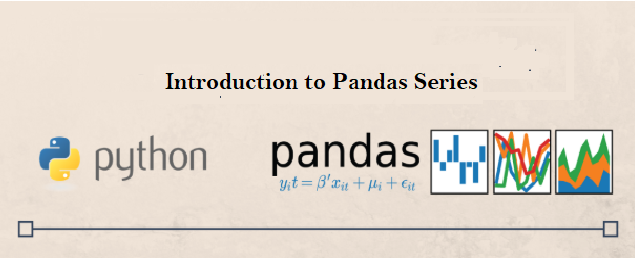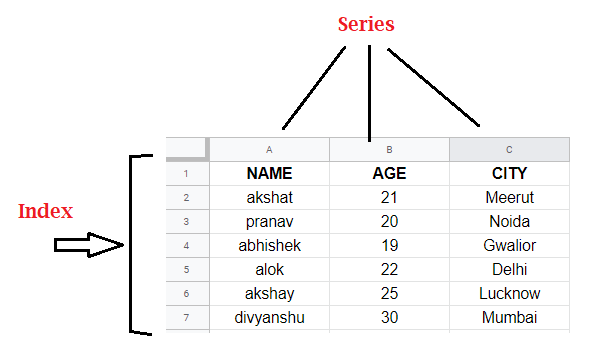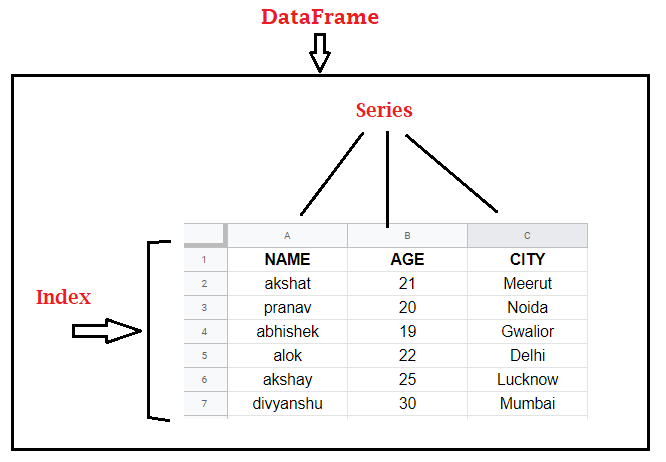×

Search anything:

# Use Series in Pandas

#### Software Engineering PythonGet this book -> Problems on Array: For Interviews and Competitive Programming

Reading time: 30 minutes | Coding time: 10 minutes

In this post, we will look at Series, which is present in the pandas library. It is very popular among python users due to its vast functionality and usability. We will dive straight to what series is, what are its uses along with some basic coding implementation.# Introduction

Before understanding what Series is, we must look at the pandas first. As stated on pandas homepage-
Pandas is an open-source, BSD-licensed library providing high-performance, easy-to-use data structures and data analysis tools for the Python programming language. It is very easy to install pandas with simple pip command typed in command prompt(cmd)-

``````pip install pandas
``````

You are most likely to encounter pandas whenever you're dealing with any data analysis or machine learning using python. Now, after having a good intuition about pandas lets look at the Series.

Pandas Series is a one-dimensional labeled array capable of holding data of any type.
The data types can be integer, string, float, python objects and many more. Series is used for storing some information.

In simple words, Series can be inferred as a column in an excel sheet. Below, we can see we have different columns like Name, Age and City,. Each of them represents a Series. And, on the left, we can see some numbers which are index. By default, these are number but they can be of other data types also.The axis labels are collectively called index. Labels need not be unique but must be a hashable type. The object supports both integer and label-based indexing and provides a host of methods for performing operations involving the index.

Now, we will look at the various parameters of Series constructor and some basic operations on Series.

• data - contains the data to be stored in Series | array-like, Iterable, dict, or scalar value
• index - values for index | must be hashable & of same length as data | Default(if no index passed)- np.arange(n) | Non-unique index values also allowed | array-like or Index (1d)
• dtype - data type for the output Series | str, numpy.dtype, or ExtensionDtype, Optional(inferred from data)
• copy - Copy the input data | bool, Default-False

## 1. Creating an empty series

``````import pandas as pd
import numpy as np
ser = pd.Series()
print(ser)
``````

OUTPUT:

``````Series([], dtype: float64)
``````

Since, we've not passed any data, the output is an empty Series.

## 2. Creating series using numpy array

``````data = np.array(['a','k','s','h','a','t'])
ser = pd.Series(data)
print(ser)
``````

OUTPUT:

``````0    a
1    k
2    s
3    h
4    a
5    t
dtype: object
``````

Note, that the dtype in output is object since we have passed string as our data. And, index is automatically determined by pandas.

## 3. Creating series using list

``````data = [10,15,20,25]
ser = pd.Series(data)
print(ser)
``````

OUTPUT:

``````0    10
1    15
2    20
3    25
dtype: int64
``````

Note, that the dtype in output is int64 since we have integers as our data.

## 4. Creating series using dictionary

``````data = {'a' : 2, 'b' : 4, 'c' : 6}
ser = pd.Series(data)
print(ser)
``````

OUTPUT:

``````a    2
b    4
c    6
dtype: int64
``````

## 5. describe() method of Series

``````data = [-1,10,15,20,25,100]
ser = pd.Series(data)
print(ser.describe())
``````

OUTPUT:

``````count      6.000000
mean      28.166667
std       36.306565
min       -1.000000
25%       11.250000
50%       17.500000
75%       23.750000
max      100.000000
dtype: float64
``````

describe() method returns the descriptive statistics of our data. In case of object data, it will return-

count - total number of values
unique - number of unique values
top - most frequent value
freq - frequency of most frequent value

## 6. Accessing element of Series

``````data = list(['akshat','bob','alexa','akansha'])
ser = pd.Series(data)
print(ser)
``````

OUTPUT-

``````alexa
``````

Code:

``````print(ser[0:2])
``````

OUTPUT-

``````0    akshat
1       bob
dtype: object
``````

## 7. Accessing element using loc and iloc

``````data = list(['akshat','bob','alexa','akansha'])
ser = pd.Series(data,index=[100,200,300,400])
print(ser.loc)
``````

OUTPUT-

``````akansha
``````

Code:

``````print(ser.iloc[:3]) # get first 3 elements
``````

OUTPUT-

``````100    akshat
200       bob
300     alexa
dtype: object
``````

ser.loc returns element with index 400 and ser.iloc[:3] returns first 3 elements

## 8. Checking for null values, getting maximum and minimum and index of maximum element

``````data = [-1,10,15,100,20,25,np.nan]
ser = pd.Series(data)

print('null value present:',ser.isnull().any())

ser.dropna(inplace=True)

print('maximum value is   ',ser.max())
print('minimum value is   ',ser.min())
print('index with maximum value ', ser.argmax())
``````

OUTPUT-

``````null value present: True
maximum value is    100.0
minimum value is    -1.0
index with maximum value  3
``````

## 9. Arithmetic operations

``````data1 = [0,2,4,6,8]
data2 = [1,3,5,7,9]

ser1 = pd.Series(data1)
ser2 = pd.Series(data2)

print(ser1+ser2)
``````

OUTPUT-

``````adding two series

0     1
1     5
2     9
3    13
4    17
dtype: int64
``````

Similarly, we can perform subtraction, multiplication and division.

## 10. Using apply() method to call a custom query function

Suppose, we want to get all odd numbers from a given series. Then, one possible solution is to use apply() method which requires you to pass some function that will be applied to every element of Series.

``````data = [4,16,10,15,100,20,25]
ser = pd.Series(data)
print(ser[ser.apply(lambda x : x%2!=0)])
``````

OUTPUT-

``````3    15
6    25
dtype: int64
``````

Here ser.apply(lambda x : x%2!=0) will return a boolean array-

``````0    False
1    False
2    False
3     True
4    False
5    False
6     True
dtype: bool<br>
``````

ser[ser.apply(lambda x : x%2!=0)] will return those elements where it will find True

## Conclusion

In this post, we looked at pandas Series and some of its basic operations.
There exist, a whole bunch of attributes and methods of Series which can't be covered in a single post, so you can read more through the pandas official documentation :)

Although, Series can be not only be studied individually, since in real life, the data consists of multiple columns/attributes which are stored as pandas DataFrame, so multiple columns are also studied together. A DataFrame is considered as a collection of Series.#### Akshat Maheshwari

Machine Learning Developer, Intern at OpenGenus | Integrated B. Tech and M. Tech student at ABV-Indian Institute of Information Technology and Management (2016 to 2021)

Use Series in Pandas